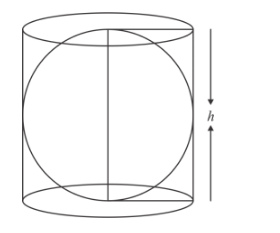# A right circular cylinder of radius r and height`
Question:

A right circular cylinder of radius r and height h (h = 2r) just encloses a sphere of diameter

(a) h

(b) r

(c) 2r

(d) 2h

Solution:Height = h

= 2r

Since, the sphere fitted the cylinder.

i.e., diameter of sphere = height of cylinder.Hence, the correct answer is choice (c).